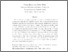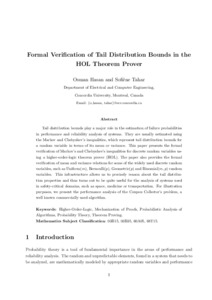Title:

# Formal verification of tail distribution bounds in the HOL theorem prover

Hasan, Osman and Tahar, Sofiène (2009) Formal verification of tail distribution bounds in the HOL theorem prover. Mathematical Methods in the Applied Sciences, 32 (4). pp. 480-504. ISSN 01704214Preview
Text (application/pdf)
formal_verification_of_tail_distribution.pdf - Accepted Version
212kB

Official URL: http://dx.doi.org/10.1002/mma.1055

## Abstract

Tail distribution bounds play a major role in the estimation of failure probabilities in performance and reliability analysis of systems. They are usually estimated using Markov's and Chebyshev's inequalities, which represent tail distribution bounds for a random variable in terms of its mean or variance. This paper presents the formal verification of Markov's and Chebyshev's inequalities for discrete random variables using a higher-order-logic theorem prover. The paper also provides the formal verification of mean and variance relations for some of the widely used discrete random variables, such as Uniform(m), Bernoulli(p), Geometric(p) and Binomial(m, p) random variables. This infrastructure allows us to precisely reason about the tail distribution properties and thus turns out to be quite useful for the analysis of systems used in safety-critical domains, such as space, medicine or transportation. For illustration purposes, we present the performance analysis of the coupon collector's problem, a well-known commercially used algorithm.

Divisions: Concordia University > Gina Cody School of Engineering and Computer Science > Electrical and Computer Engineering Article Yes Hasan, Osman and Tahar, Sofiène Mathematical Methods in the Applied Sciences 2009 10.1002/mma.1055 974500 ANDREA MURRAY 31 Jul 2012 20:04 18 Jan 2018 17:38
All items in Spectrum are protected by copyright, with all rights reserved. The use of items is governed by Spectrum's terms of access.

Repository Staff Only: item control pageResearch related to the current document (at the CORE website)
Back to top